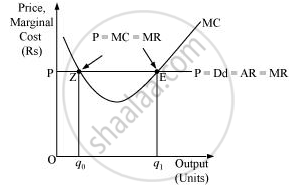Advertisement Remove all ads

# Will a Profit-maximising Firm in a Competitive Market Ever Produce a Positive Level of Output in the Range Where the Marginal Cost is Falling? Give an Explanation. - Economics

Short Note

Will a profit-maximising firm in a competitive market ever produce a positive level of output in the range where the marginal cost is falling? Give an explanation.

Advertisement Remove all ads

#### Solution

It is not possible for any perfect competitive firm to produce a positive level of output in a range where MC is falling. This is because, according to one of the conditions of profit-maximisation, MC curve should be upward sloping or the slope of MC curve should be positive at the equilibrium level of output.

Let us take an example:-

At point Z price is equal to MC, but MC is falling and is negatively sloped. For any level of output more than Oq0, the firm is facing price > MC, which implies that the profit can be maximised by increasing the output level further.Hence, the point ‘E’ is the equilibrium point, where a profit maximising firm would operate and produce Oq1 units of output and its profit will be maximised.

Is there an error in this question or solution?
Advertisement Remove all ads

#### APPEARS IN

NCERT Class 12 Economics Introductory Microeconomics
Chapter 4 The Theory Of The Firm Under Perfect Competition
Exercise | Q 9 | Page 68
Advertisement Remove all ads
Advertisement Remove all ads
Share
Notifications

View all notifications

Forgot password?